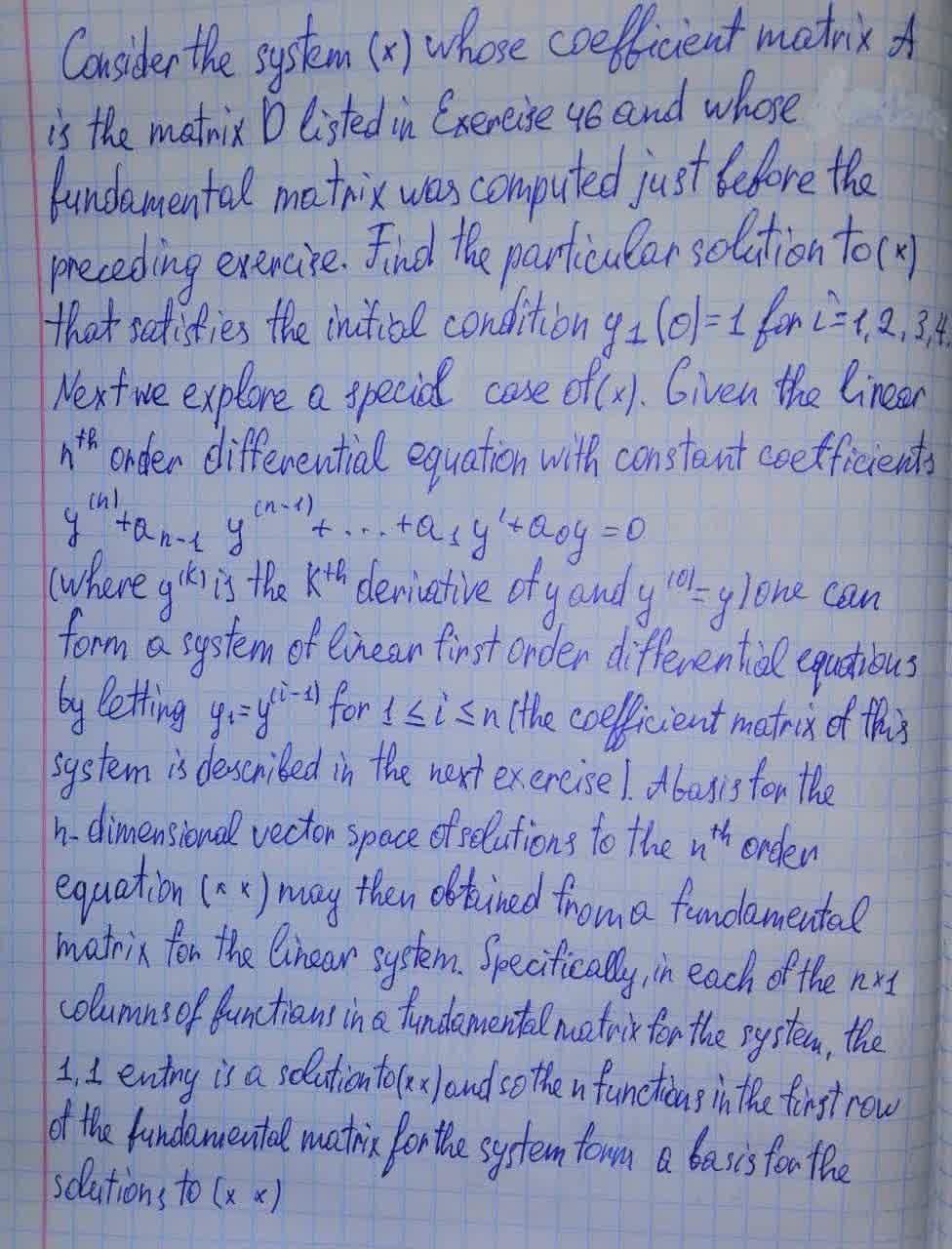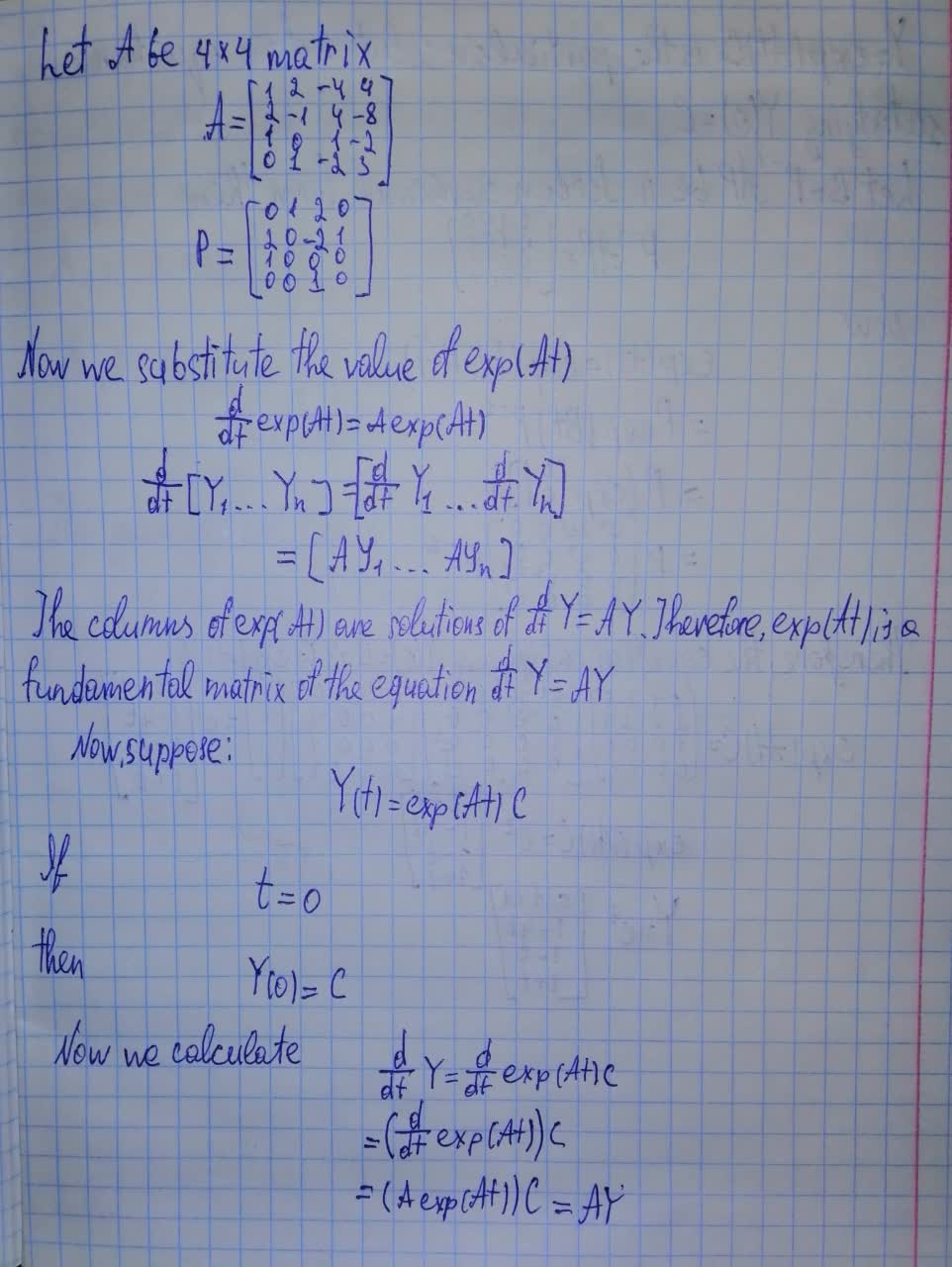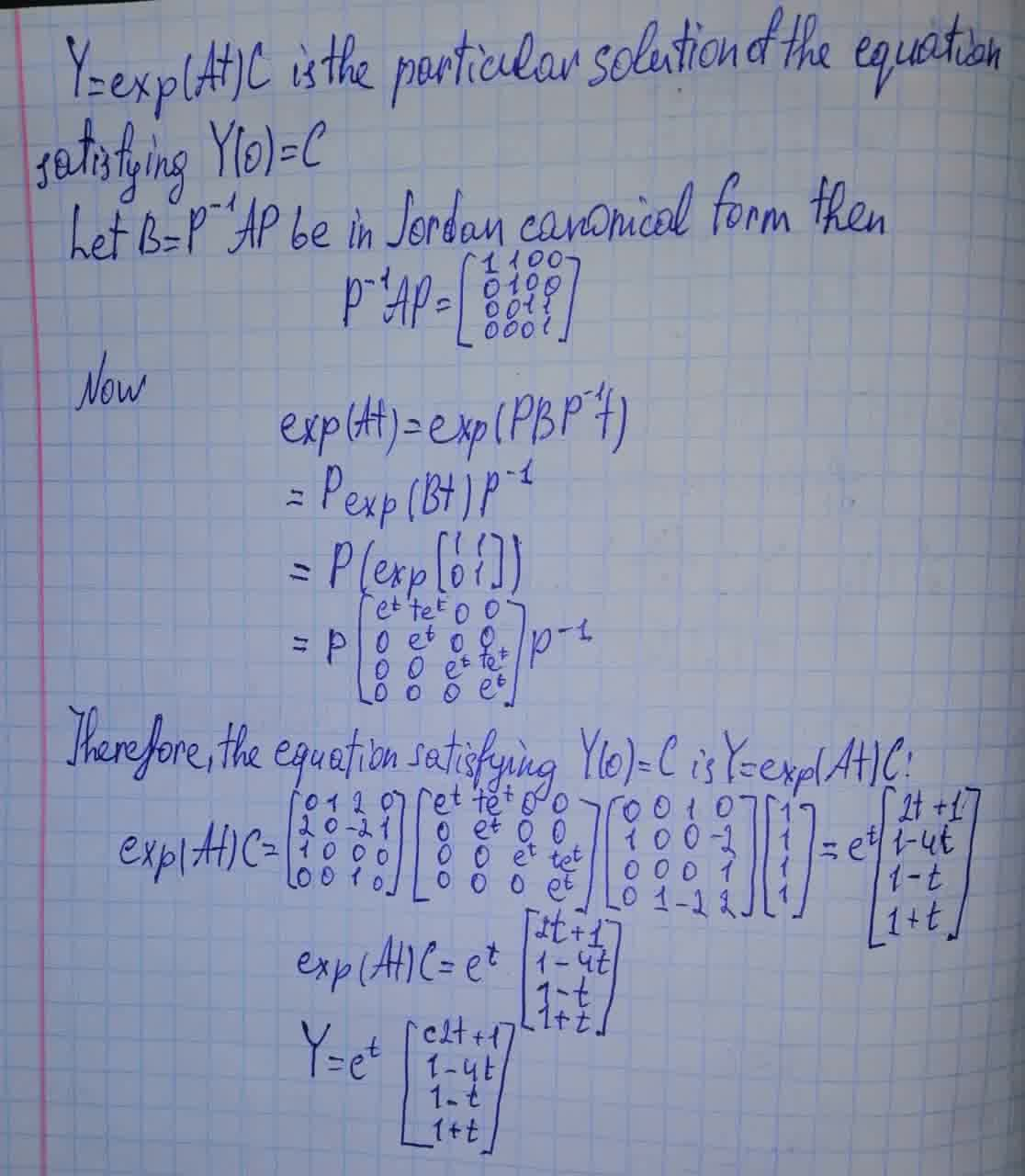Consider the system whose coefficient matrix A is the matrix D listed in and whose fundamental matrix was computed just before the preceding exercise.hexacordoK 2021-08-14 Answered
Consider the system (*) whose coefficient matrix A is the matrix D listed in Exercise 46 and whose fundamental matrix was computed just before the preceding exercise.• Questions are typically answered in as fast as 30 minutes

Solve your problem for the price of one coffee

• Math expert for every subject
• Pay only if we can solve itLiyana Mansell

Explanation in the photo below